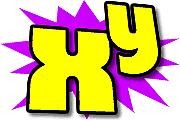# Product 3108

The product of 3 numbers is 42. The first is 1.5 times larger than the second number, and the third is 3.5 times larger than the second number. What numbers are these?

a =  3
b =  2
c =  7

### Step-by-step explanation:Did you find an error or inaccuracy? Feel free to write us. Thank you!

Tips for related online calculators
Do you have a linear equation or system of equations and looking for its solution? Or do you have a quadratic equation?